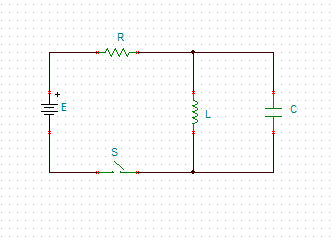# In the circuit the battery emf is 75 V, the resistance R is 160 ohm, and the capacitance C is...

## Question:

In the circuit the battery emf is {eq}75 V, {/eq} the resistance {eq}R {/eq} is {eq}160 \Omega {/eq} , and the capacitance {eq}C{/eq} is {eq}0.500 \mu F.{/eq} The switch {eq}S {/eq} is closed for a long time, and no voltage is measured across the capacitor. After the switch is opened, the potential difference across the capacitor reaches a maximum value of {eq}150 V. {/eq} What is the value of the inductance {eq}L?{/eq} _ H## RLC circuit

We know that when capacitor becomes fully charged then it behave as open circuit and when it is uncharged state then it behave as short circuit. While it is just reverse for the inductor that it will behave as short circuit after getting fully charged.

Once the capacitor is fully charged then it will behave as open circuit and inductor will behave as short circuit.

Therefore on opening the swith it...

Become a Study.com member to unlock this answer! Create your account